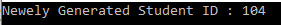In this article, I am going to discuss ADO.NET Using Stored Procedure in detail. Please read our previous article, where we discussed ADO.NET DataSet Architecture. At the end of this article, you will understand how to call a stored procedure without parameter as well as how to call a stored procedure from ADO.NET using both inputs as well as the output parameter.

Before understanding how to call a stored procedure, let us first understand what is a stored procedure.

##### What is a Stored Procedure in SQL?

A Stored Procedure in SQL is a database object which contains pre-compiled SQL Statements. In simple words, we can also say that the Stored Procedures are a block of code that is designed to perform a specific task whenever it is called. Please click here to learn SQL Server Stored Procedure in detail.

##### Example to understand ADO.NET using Stored Procedure:

We are going to use the following student table in this demo to understand the concept of ADO.NET using Stored Procedure.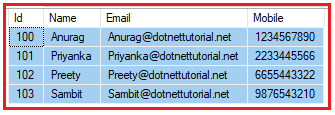Please use below SQL Script to create and populate the database StudentDB and table Student with the required sample data.

```CREATE DATABASE StudentDB;
GO

USE StudentDB;
GO

CREATE TABLE Student(
[Id] [int] IDENTITY(100,1) PRIMARY KEY,
[Name] [varchar](100) NULL,
[Email] [varchar](50) NULL,
[Mobile] [varchar](50) NULL,
)
GO

INSERT INTO Student VALUES ('Anurag','Anurag@dotnettutorial.net','1234567890')
INSERT INTO Student VALUES ('Priyanka','Priyanka@dotnettutorial.net','2233445566')
INSERT INTO Student VALUES ('Preety','Preety@dotnettutorial.net','6655443322')
INSERT INTO Student VALUES ('Sambit','Sambit@dotnettutorial.net','9876543210')```
##### Stored Procedure without a parameter:

So, the first thing is always first. Let us create a stored procedure that will not take any input parameter but will return all the records from the student table. Please use below SQL Script to create the stored procedure.

```CREATE PROCEDURE spGetStudents
AS
BEGIN
SELECT Id, Name, Email, Mobile
FROM Student
END```
##### How to call a stored procedure using C# ADO.NET.

Let us see the step by step procedure to call the above-stored procedure. Please have a look at the below image. So, what you need to do is, first create an instance of command object and then specify the commandTest property value as the stored procedure name and the most important point is you need to specify the command type as Stored Procedure.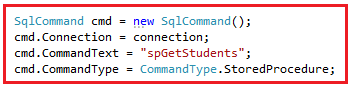You can also use the other overloaded constructor of Command class as shown in the below image. As you can see, here we are passing the stored procedure name and the connection object to the constructor of the command object and then specify the command type as a Stored procedure.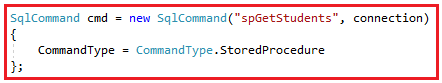###### The complete example is given below.
```using System;
using System.Data;
using System.Data.SqlClient;

{
class Program
{
static void Main(string[] args)
{
try
{
string ConnectionString = "data source=.; database=StudentDB; integrated security=SSPI";
using (SqlConnection connection = new SqlConnection(ConnectionString))
{
SqlCommand cmd = new SqlCommand("spGetStudents", connection)
{
CommandType = CommandType.StoredProcedure
};

connection.Open();
{
Console.WriteLine(sdr["Id"] + ",  "+sdr["Name"] + ",  " + sdr["Email"] + ",  " + sdr["Mobile"]);
}
}
}
catch (Exception e)
{
Console.WriteLine("OOPs, something went wrong.\n" + e);
}
}
}
}```

Output: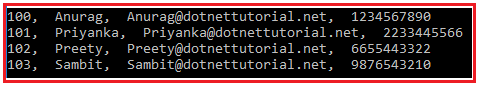##### Stored procedure with Input Parameter:

Now, we will see how to call a stored procedure with an input parameter. So, please use the below SQL Script to create the stored procedure which will return the student details by id. Here, student id is the input parameter and that parameter value we need to pass while calling this stored procedure.

```CREATE PROCEDURE spGetStudentById
(
@Id INT
)
AS
BEGIN
SELECT Id, Name, Email, Mobile
FROM Student
WHERE Id = @Id
END```
##### How to call a stored procedure with an input parameter in C# ADO.NET?

In order to understand how to call a stored procedure with an input parameter, please have a look at the following image. We already discussed the command object. The point that you need to focus on here is the SqlParameter object. As you can see here we are creating an instance of SqlParameter object and then setting the parameter name, the data type, value, and the direction of the parameter.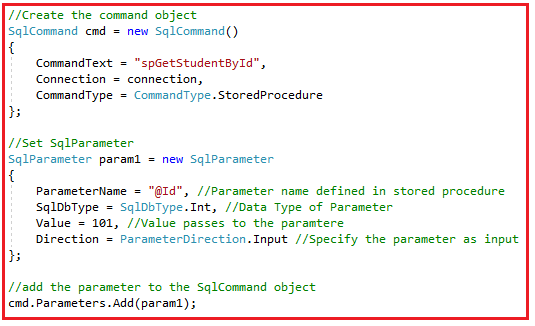###### The complete example is given below.
```using System;
using System.Data;
using System.Data.SqlClient;

{
class Program
{
static void Main(string[] args)
{
try
{
string ConnectionString = "data source=.; database=StudentDB; integrated security=SSPI";
using (SqlConnection connection = new SqlConnection(ConnectionString))
{

//Create the command object
SqlCommand cmd = new SqlCommand()
{
CommandText = "spGetStudentById",
Connection = connection,
CommandType = CommandType.StoredProcedure
};

//Set SqlParameter
SqlParameter param1 = new SqlParameter
{
ParameterName = "@Id", //Parameter name defined in stored procedure
SqlDbType = SqlDbType.Int, //Data Type of Parameter
Value = 101, //Value passes to the paramtere
Direction = ParameterDirection.Input //Specify the parameter as input
};

//add the parameter to the SqlCommand object

connection.Open();
{
Console.WriteLine(sdr["Id"] + ",  "+sdr["Name"] + ",  " + sdr["Email"] + ",  " + sdr["Mobile"]);
}
}
}
catch (Exception e)
{
Console.WriteLine("OOPs, something went wrong.\n" + e);
}
}
}
}```

Output: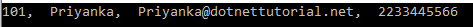##### Stored Procedure with both Input and Output Parameter:

In our previous example, we understand how to call a stored procedure with an input parameter. Now, let us see how to call a stored procedure with both input and output parameters. So, please use below SQL Script to create the stored procedure with both input and output parameters.

```CREATE PROCEDURE spCreateStudent
(
@Name VARCHAR(100),
@Email VARCHAR(50),
@Mobile VARCHAR(50),
@Id int Out
)
AS
BEGIN
INSERT INTO Student VALUES (@Name,@Email,@Mobile)
SELECT @Id = SCOPE_IDENTITY()
END```

As you can see the above-stored procedure, takes four parameters (3 input + 1 output). The above-stored procedure is very simple, takes the Student Name, Email and Mobile and inserts into the Student table. As we created the student table with Id as an Identity column, so, we don’t require to pass the id value in the insert statement. But what we want here is we need to return the newly created student id and this is where the output parameter comes into the picture. Here, we set the output parameter value with the newly generated student id.

##### How to call a stored procedure with both input and output parameter in C#?

In order to understand how to call a stored procedure with both input and output parameters, please have a look at the following image. As you can see, while defining the Output Parameter you need to specify the parameter direction as Output and you don’t require to set the value property.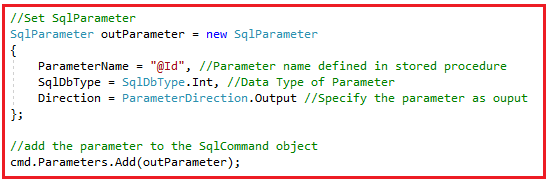Note: By default the parameter direction is Input.

##### The complete code is given below.
```using System;
using System.Data;
using System.Data.SqlClient;

{
class Program
{
static void Main(string[] args)
{
try
{
string ConnectionString = "data source=.; database=StudentDB; integrated security=SSPI";
using (SqlConnection connection = new SqlConnection(ConnectionString))
{
//Create the command object
SqlCommand cmd = new SqlCommand()
{
CommandText = "spCreateStudent",
Connection = connection,
CommandType = CommandType.StoredProcedure
};

//Set SqlParameter
SqlParameter param1 = new SqlParameter
{
ParameterName = "@Name", //Parameter name defined in stored procedure
SqlDbType = SqlDbType.NVarChar, //Data Type of Parameter
Value = "Test",
Direction = ParameterDirection.Input //Specify the parameter as input
};

//add the parameter to the SqlCommand object

//Another approach to add input parameter

//Set SqlParameter
SqlParameter outParameter = new SqlParameter
{
ParameterName = "@Id", //Parameter name defined in stored procedure
SqlDbType = SqlDbType.Int, //Data Type of Parameter
Direction = ParameterDirection.Output //Specify the parameter as ouput
};

//add the parameter to the SqlCommand object

connection.Open();
cmd.ExecuteNonQuery();

Console.WriteLine("Newely Generated Student ID : " + outParameter.Value.ToString());
}
}
catch (Exception e)
{
Console.WriteLine("OOPs, something went wrong.\n" + e);
}